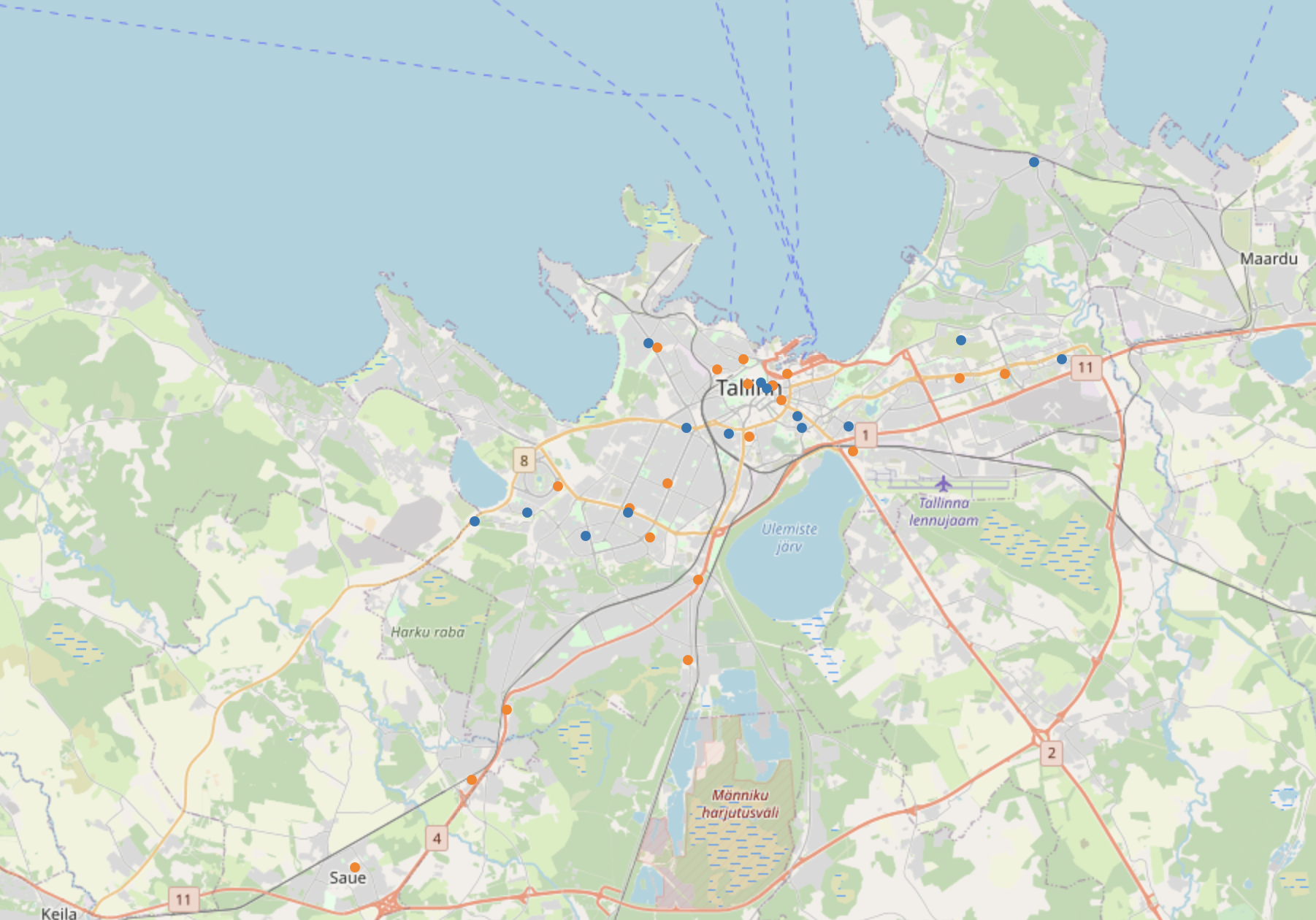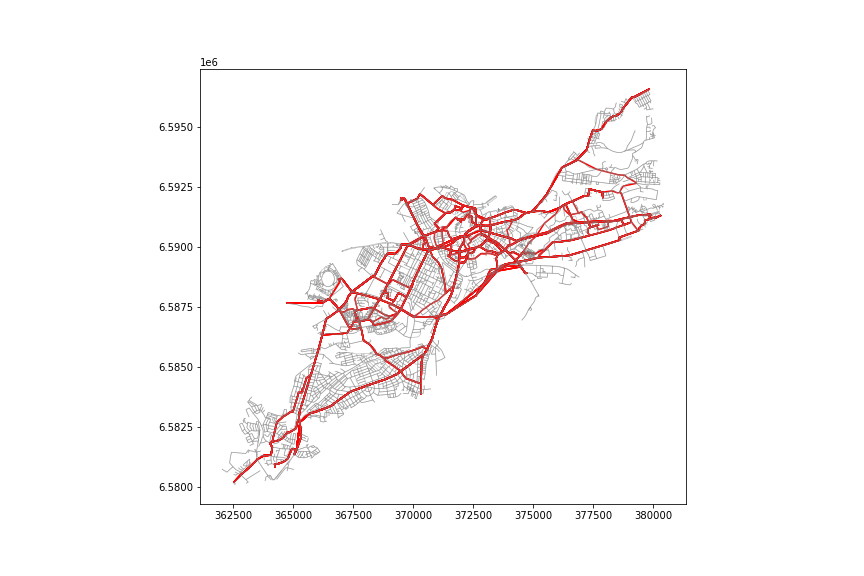# Basics of Python x GIS (Part 3)

The first is here Part 2 is here University of Helsinki Teaching Materials We will summarize the answers and supplements for Week5-Week6.

Week5

### 5-1 Creating a static map

In Week5, the original notebook does not exist, so you will be required to read the README on Github, select the appropriate material yourself, and visualize the map.

Week6

### 6-1 Guess the location of the store

In the first half of Week6, we will use OSM (Open Street Map) to estimate the departure and arrival points of the movement data given in advance. The `mplleaflet` library allows you to plot shapes created with` matplotlib` on an interactive map. (For `mplleaflet`, see This article .)` mplleaflet` cannot save images by itself. It is necessary to save the image using a screenshot or the like.

``````#Data reading
origin = pd.read_csv('data/origins.csv') #Point of departure
#Pointing
make_point = lambda row:Point(row['x'], row['y'])
origin['geometry'] = origin.apply(make_point, axis=1)
dest['geometry'] = dest.apply(make_point, axis=1)
origin_geo = gpd.GeoDataFrame(origin, geometry='geometry')
dest_geo = gpd.GeoDataFrame(dest, geometry='geometry')
#Coordinate system definition and transformation
rigin_geo.crs = CRS.from_epsg(3857)
dest_geo.crs = CRS.from_epsg(3857)
origin_geo = origin_geo.to_crs(CRS.from_epsg(3857))
dest_geo = dest_geo.to_crs(CRS.from_epsg(3857))
#plot
fig, ax = plt.subplots(figsize=(12,8))
origin_geo.plot(ax=ax)
dest_geo.plot(ax=ax)
mplleaflet.show() #Combine with map
``````You can see that points are gathered near Tallinn, the capital of Estonia. Let's check by geocoding.

### 6-2 Tallinn network analysis

The goal is to find the route / distance between the starting point and the arrival point obtained in 6-1. (1) Convert to a graph using the ʻosmx`library based on OSM road data ② Approximate the starting point / arrival store to the nearest node in the graph ③ Create the shortest route between nodes with`networkx` and find its length Work in this order.

``````#Creating a convex hull that includes all points
merged = pd.concat([origin, dest])
make_p = lambda row: (row['geometry'].x, row['geometry'].y)
merged['p'] = merged.apply(make_p, axis=1)
list_point = merged['p'].values.tolist()
multi = MultiPoint(list_point)
extent = multi.convex_hull
#Graph extraction
import osmnx as ox
graph = ox.graph_from_polygon(extent, network_type='drive')
#Create nodes and links from graphs
nodes_proj, edges_proj = ox.graph_to_gdfs(graph_proj, nodes=True, edges=True)
#Approximation of points to nodes
graph_proj = ox.project_graph(graph)
orig_nodes = []
for orig in origin_geo['geometry']:
orig_xy = (orig.y, orig.x)
orig_node = ox.get_nearest_node(graph, orig_xy)
orig_nodes.append(orig_node)
dest_nodes = []
for des in dest_geo['geometry']:
dest_xy = (des.y, des.x)
dest_node = ox.get_nearest_node(graph, dest_xy)
dest_nodes.append(dest_node)
#Create minimum path
route_lines = []
route_lengths=[]
for i in orig_nodes:
for j in dest_nodes:
if i==j: #Excludes if the starting and arriving points are close to the same point
continue
route = nx.shortest_path(G=graph, source=i, target=j, weight='length')
route_nodes = nodes_proj.loc[route]
route_line = LineString(list(route_nodes.geometry.values))
route_lines.append(route_line)
route_lengths.append(route_line.length)
route_geom = pd.DataFrame([route_lines, route_lengths]).T
route_geom.columns = ['geometry', 'route_dist']
route_geom = gpd.GeoDataFrame(route_geom,geometry='geometry', crs=edges_proj.crs)
#Shortest and longest distance
mini = route_geom['route_dist'].min()
maxx = route_geom['route_dist'].max()
#Visualization
fig, ax = plt.subplots(figsize=(12,8))
route_geom.plot(ax=ax, color='red')
edges_proj.plot(ax=ax, color='gray', alpha=0.5, linewidth=0.7)
fig.savefig('network.png')
``````If you paint all the routes, it will look like the figure above. For network analysis, it seems that you can try various things using Python rather than using GIS.